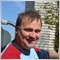# How to calculate current profit in pips1052

Hello to all,

I've seen several posts but no one has helped me ....
I made an EA that open several orders, I wish to calculate to the whole order with a specific magic number, the algebraic sum of profit or loss in pips .
I set a cycle for scanning all orders, and for each one:
And if sell between OpenOrserPrice-Bid and then converting in pips . Then at the end summing  pips on each order.
This calculation is carried out call this function from OnTick function but I have seen that calculation is wrong in something. Can you help me ?20214

Francesco Fava:

If buy I calculated the difference between Ask-OpenOrderPrice And if sell between OpenOrserPrice-Bid and then converting in pips . Then at the end summing  pips on each order.

This calculation is carried out call this function from OnTick function but I have seen that calculation is wrong in something. Can you help me ?
1. You've got it correct
2. "wrong in something" is meaningless - just like saying the car doesn't work. Doesn't start, won't go in gear, no electrical, missing the key, flat tires - meaningless. We can't see your broken code. There are no mind readers here and our crystal balls are cracked.1052

whroeder1:
1. You've got it correct
2. "wrong in something" is meaningless - just like saying the car doesn't work. Doesn't start, won't go in gear, no electrical, missing the key, flat tires - meaningless. We can't see your broken code. There are no mind readers here and our crystal balls are cracked.
I used marketinfo function in this way:
OnInit:
if(Digits == 3 || Digits == 5) point_compat = 10;

And

double CheckPips()
{

int total = OrdersTotal();
double diff=0;
for (int cnt =total-1 ; cnt>=0 ; cnt--)
{
if(OrderMagicNumber()==magico) {
double diff1=OrderOpenPrice();
double DiffPips = (NormalizeDouble(((Ask - diff1)/MarketInfo(Symbol(),MODE_POINT)),(int)MarketInfo(Symbol(),MODE_DIGITS)))/point_compat;
diff=diff+DiffPips;
}

if (OrderType()==OP_SELL) {
double diff1=OrderOpenPrice();
double DiffPips = (NormalizeDouble(((diff1 - Bid)/MarketInfo(Symbol(),MODE_POINT)),(int)MarketInfo(Symbol(),MODE_DIGITS)))/point_compat;
diff=diff+DiffPips;
}
}

}
double tot=NormalizeDouble(diff,2);
ScriviSuChart("ObjName1","cumulated pips: " + (string)tot,20);
}1052

I used this function1787

```   for (int cnt = OrdersTotal() - 1; cnt >= 0; cnt--)
{
if (OrderSelect(cnt, SELECT_BY_POS, MODE_TRADES) && OrderSymbol() == Symbol())
{
if (OrderMagicNumber() == MagicS)
{
gProfit += OrderProfit(); // add Swap and Commission if you care
gOrdersCount += 1;
gLots += OrderLots();

gWeightedAverage += OrderLots() * OrderOpenPrice();
}
}
}
if (gLots > 0) gWeightedAverage /= gLots;```

make sure you init the vars to 0

at the end of this you will all your heart desires -> Profit, Ordercount, Total Lot Size and and average order open price.

you now can use this average order open price similar to how you used diff1 before.  Example = ( (gWeightedAverage - Bid) / Point) / Point_Compat.  and then normalize it1052

Cornelis Duiker:

make sure you init the vars to 0

at the end of this you will all your heart desires -> Profit, Ordercount, Total Lot Size and and average order open price.

you now can use this average order open price similar to how you used diff1 before.  Example = ( (gWeightedAverage - Bid) / Point) / Point_Compat.  and then normalize it

But I have to calculate weighted average separately for buy and sell orders ? Because you have said Example = ( (gWeightedAverage - Bid) / Point) / Point_Compat and so you placed Bid .... This if for sell orders1052

I will have to calculate both
( (gWeightedAverage - Bid) / Point) / Point_Compat

And

( (Ask - gWeightedAverage - ) / Point) / Point_Compat

How to calculate PERFECTLY the number of profit pips of all opened trades ?1052

I used EquitySentry and my EA and the floating profit / loss doesn't correspond11676

Francesco Fava:
I used EquitySentry and my EA and the floating profit / loss doesn't correspond

Your function seems correct to me. Maybe is there some commissions on your account ?

Then do not confuse points with pips.

`  double DiffPips = (NormalizeDouble(((Ask - diff1)/MarketInfo(Symbol(),MODE_POINT)),(int)MarketInfo(Symbol(),MODE_DIGITS)))/_Point;`